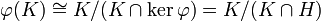Local finiteness is extension-closed

This article gives the statement, and possibly proof, of a group property (i.e., locally finite group) satisfying a group metaproperty (i.e., extension-closed group property)
View all group metaproperty satisfactions | View all group metaproperty dissatisfactions |Get help on looking up metaproperty (dis)satisfactions for group properties
Get more facts about locally finite group |Get facts that use property satisfaction of locally finite group | Get facts that use property satisfaction of locally finite group|Get more facts about extension-closed group property

Statement

Statement with symbols

Suppose$G$ is a group with a normal subgroup$H$ such that both$H$ and$G/H$ are locally finite groups: any finitely generated subgroup of$H$ is finite, and any finitely generated subgroup of$G/H$ is finite. Then,$G$ is itself locally finite.

Facts used

1. Schreier's lemma: In its factual form, this states that any subgroup of finite index in a finitely generated group is again finitely generated.
2. Finiteness is extension-closed
3. First isomorphism theorem

Proof

Given: A group$G$ with a normal subgroup$H$ such that both$H$ and$G/H$ are locally finite. Let$\varphi:G \to G/H$ be the quotient map.A finite subset$A$ of$G$ with$K = \langle A \rangle$.

To prove:$K$ is finite.

Proof:

Step no. Assertion/construction Facts used Given data used Previous steps used Explanation
1$\varphi(K)$ is a finite subgroup of$G/H$$G/H$ is locally finite Since$A$ is finite,$\varphi(A)$ is finite. By local finiteness of$G/H$,$\langle \varphi(A) \rangle$ is a finite subgroup of$G/H$. We have$\langle \varphi(A) \rangle = \varphi(\langle A \rangle) = \varphi(K)$, so$\varphi(K)$ is finite.
2$K \cap H$ is a normal subgroup of finite index in$K$ Fact (3) We have$\varphi(K) \cong K/(K \cap \ker \varphi) = K/(K \cap H)$. Since the left side is finite, we conclude that$K \cap H$ has finite index in$K$.
3$K \cap H$ is finitely generated Fact (1)$A$ is finite Step (2)$A$ is finite, so$K = \langle A \rangle$ is finitely generated, and by step (2),$K \cap H$ has finite index in$K$. Thus, by fact (1),$K \cap H$ is also a finitely generated group.
4$K \cap H$ is finite$H$ is locally finite Step (3)$K \cap H$ is a finitely generated subgroup of$H$. Local finiteness of$H$ forces$K \cap H$ to be finite.
5$K$ is finite Fact (2) Steps (2), (4) By step (4),$K \cap H$ is finite, and by step (2),$H/(H \cap N)$ is finite. Thus, by fact (2),$K$ is finite.
This proof uses a tabular format for presentation. Provide feedback on tabular proof formats in a survey (opens in new window/tab) | Learn more about tabular proof formats|View all pages on facts with proofs in tabular format